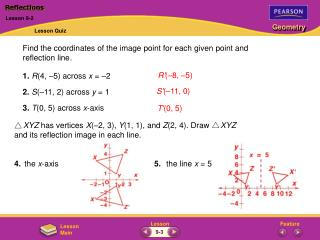DownloadDownload PresentationReflections

# Reflections

Télécharger la présentation## Reflections

- - - - - - - - - - - - - - - - - - - - - - - - - - - E N D - - - - - - - - - - - - - - - - - - - - - - - - - - -
##### Presentation Transcript

1. XYZ has vertices X(–2, 3), Y(1, 1), and Z(2, 4). Draw XYZ and its reflection image in each line. 4. the x-axis 5. the line x = 5 Reflections Lesson 9-2 Lesson Quiz Find the coordinates of the image point for each given point and reflection line. 1. R(4, –5) across x = –2 2. S(–11, 2) across y = 1 3. T(0, 5) across x-axis R'(–8, –5) S'(–11, 0) T'(0, 5) 9-3

2. Rotations Lesson 9-3 Check Skills You’ll Need (For help, go to the Skills Handbook, page 746 and Lesson 1-7.) Use a protractor to draw an angle with the given measure. 1. 120 2. 90 3. 72 4. 60 5. 45 6. 36 7. Draw a segment, AB. Then construct A'B' congruent to AB. Check Skills You’ll Need 9-3

3. Rotations Lesson 9-3 Check Skills You’ll Need Solutions For problems 1–7, check students’ work. 9-3

4. Rotations Lesson 9-3 To describe a rotation, you need to know the center of rotation (a point), the angle of rotation (a positive number of degrees), and whether the rotation is clockwise or counterclockwise. Unless stated otherwise, rotations in this book are counterclockwise. 9-3

5. Rotations Lesson 9-3 You can use the following two rules to rotate a figure through x° about a point R: • The image of R is itself (that is, R’ = R). • For any point V, RV’ = RV and mVRV’ = x. 9-3

6. Rotations Lesson 9-3 The center of a regular polygon is the point equidistant from its vertices. Segments that connect the center to the vertices divide the polygon into congruent triangles. You can use this fact to find rotation images of regular polygons. 9-3

7. Copy LOB, and draw its image under a 60° rotation about C. Step 1: Use a protractor to draw a 60° angle at vertex C with one side CO. Rotations Lesson 9-3 Additional Examples Drawing a Rotation Image 9-3

8. Step 2: Use a compass to construct COCO. Step 3: Locate L and B in a similar manner. Then draw L O B . Rotations Lesson 9-3 Additional Examples (continued) Quick Check 9-3

9. a. Because 360° ÷ 6 = 60°, each central angle of ABCDEF measures 60. A 240° counterclockwise rotation about center M moves point B across four triangles. The image of point B is point D. b.AMF is equilateral, so AFM has measure 180 ÷ 3 = 60. A 60° rotation of AMF about point F would superimpose FM on FA, so the image of M under a 60° rotation about point F is point A. Rotations Lesson 9-3 Additional Examples Identifying a Rotation Image Regular hexagon ABCDEF is divided into six equilateral triangles. a. Name the image of B for a 240° rotation about M. b. Name the image of M for a 60° rotation about F. Quick Check 9-3

10. Rotations Lesson 9-3 Additional Examples Finding the Angle of Rotation A regular 12-sided polygon can be formed by stacking congruent square sheets of paper rotated about the same center on top of each other. Find the angle of rotation about M that maps W to B. Consecutive vertices of the three squares form the outline of a regular 12-sided polygon. 360 ÷ 12 = 30, so each vertex of the polygon is a 30° rotation about point M. You must rotate counterclockwise through 7 vertices to map point W to point B, so the angle of rotation is 7 • 30°, or 210°. Quick Check 9-3

11. Rotations Lesson 9-3 Additional Examples Compositions of Rotations Describe the image of quadrilateral XYZW for a composition of a 145° rotation and then a 215° rotation, both about point X. The two rotations of 145° and 215° about the same point is a total rotation of 145° + 215°, or 360°. Because this forms a complete rotation about point X, the image is the preimage XYZW. Quick Check 9-3

12. Copy RST and point C. Draw the image for the given transformation about point C. Label the vertices of the image. FG Rotations Lesson 9-3 Lesson Quiz 1. 75° rotation 2. composition of a 30° rotation and then a 150° rotation ABCDEFGH is a regular octagon. Name the image for the given rotation. 4. 270° rotation of DE about O 3. 135° rotation of A about O F 5. 135° rotation of B about O G 9-3